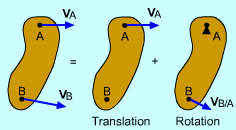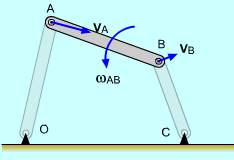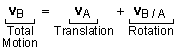Ch 5. Rigid Body General Motion Multimedia Engineering Dynamics Fixed Axis Rotation Plane Motion Velocities Zero Velocity Point Plane Motion Accelerations Multiple Gears Rot. Coord. Velocities Rot. Coord. Acceleration
 Chapter - Particle - 1. General Motion 2. Force & Accel. 3. Energy 4. Momentum - Rigid Body - 5. General Motion 6. Force & Accel. 7. Energy 8. Momentum 9. 3-D Motion 10. Vibrations Appendix Basic Math Units Basic Equations Sections Search eBooks Dynamics Fluids Math Mechanics Statics Thermodynamics Author(s): Kurt Gramoll ©Kurt GramollDYNAMICS - THEORY2D Rigid Body Motion is the
Sum of the Translation and
Rotation Velocities

Two dimensional motion includes both a translation and rotation components. To solve problems, it is usually easier to break rigid body motion into these two components.

To derive the 2D relative velocity equation, first relate two arbitrary points on a rotating body,

rB = rA + rB/A

Differentiating with respect to time gives

drB /dt = drA /dt + drB/A /dt

 vB = vA + vB/AStructure Motion

The relative velocity equation can be separated into linear motion and angular motion,The rotation equation can also be written as

vB = vA + ω × rB/A

vB = vA + ωret

Practice Homework and Test problems now available in the 'Eng Dynamics' mobile app
Includes over 400 problems with complete detailed solutions.
Available now at the Google Play Store and Apple App Store.